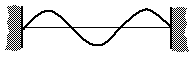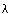Back to "Answer-This" Listing "Answer This" Questions Chapter 2 2-C. Standing wave on a string fixed at both ends Consider the diagram in the figure for a standing wave on a string 12 feet long that is tied at both ends. The wave velocity in the string is 16 ft/s. What is the wavelength?Answer: 8 feet. The wavelength is the peak to peak distance, but can also be measured in the following way: start at the left end, move past the first crest to the node (1/3 of the way along), move past the trough to the next node (2/3 of the length). The wavelength includes two consecutive antinodes and it is 2/3 of the entire string length, or 2/3 of 12 = 8 ft. The wave velocity is irrelevant to the question.   ___________________________ 2-C. Standing wave. Which mode? What frequency? Again consider the standing wave in the above figure. The length is still 12 ft and the wave velocity 16 ft/s. Which mode is this? What is the frequency of this mode? Answer: This is the 3rd harmonic or the 2nd overtone. The fundamental (or 1st harmonic) has just one antinode fitting between the two walls (and two nodes—at the ends). The first overtone (2nd harmonic) has two antinodes (and three nodes); and the second overtone (3rd harmonic) has three antinodes (four nodes) as does the figure. The frequency is wave velocity divided by wave length = (16 ft/s)/(8 ft) = 2 Hz.   ___________________________ 2-C. Standing wave. What can you change? For a standing wave on a given string a) you can have anyand any f so long as the relationship v = fholds. b) only certain wavelengths and frequencies are allowed. c) the wave velocity is totally determined by the medium. d) the wave velocity depends on the dynamic and inertial parameters. e) a and c only. f) b, c and d only. g) a, c and d only. h) none of the above. Answer: f) When you tie the ends of a string down, the wavelength must be such that there are zeros (nodes) of the wave at the ends. This means that we cannot have just any wavelength; the wavelength must be adjusted to fit into the space properly. Thus only certain wavelengths, and hence frequencies, are allowed and b) becomes true. c) and d) are also true: The wave velocity depends on the properties of the medium such as the tension of a string or its density (the dynamical and inertial properties mentioned).Thus the correct answer is f). Back to "Answer-This" Listing     © William J. Mullin